output.to from Sideway
Draft for Information Only

# Content

```Linear Approximation Ratio  Approximation Calculation     Function Approximation Problem   Error Estimation Problem   Change Determination Problem```

# Linear Approximation Ratio

The previous interpretations of a derivative focuses on the position of a point by taking the limit approaching zero. In other words, the derivative of a function at a point is the most accurate approximation to the rate of change of the function at the point. Therefore the derivative of a point can be used as the linear approximation ratio or the slope of a linear function to approximate the change in a function near the point of differentiation. The accuracy of linear approximation is high when the distance away from the derivative of a point is small, i.e. δε>Δε>0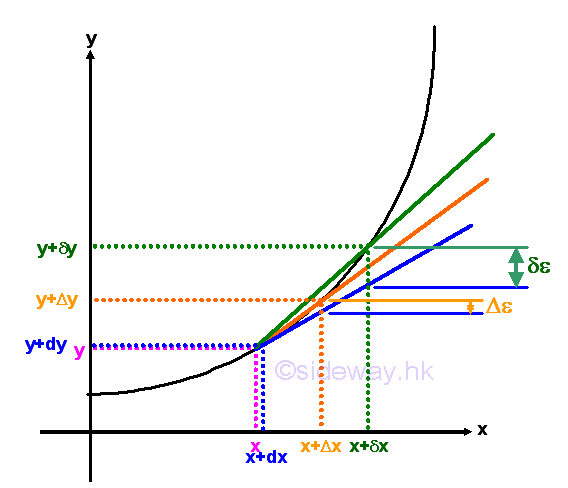## Approximation Calculation

In some practical applications, problems may be finding the change or the solutions can be approximated by a small change of the known result.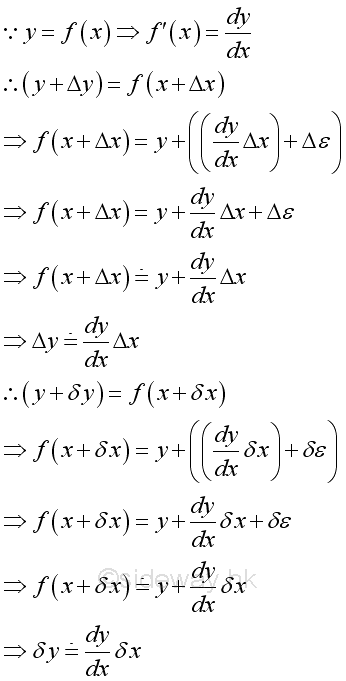### Function Approximation Problem

The value of a function of a variable can be approximated by a function of known parameter value when the difference between the variable and parameter is small.

For example, cubic root of 28. Let function y be cubic function of a variable p. A known parameter of function is 27 and the knwon solution is 3. Therefore the solution of cubic root is 3.0366 and the approximation is: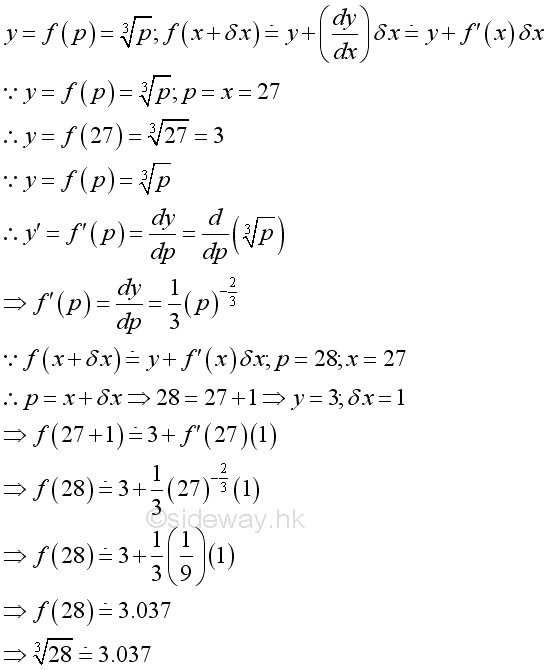### Error Estimation Problem

Measuring error is the variation in mesurements. Error is the difference between the measured value and the true value of the meausred object. The result of measuring error can be estimated by derivative.

For example, the maximum error of measuring the surfare area of a circular plate, when the measured diameter is 10 and the maximum meausring error is 0.1 is,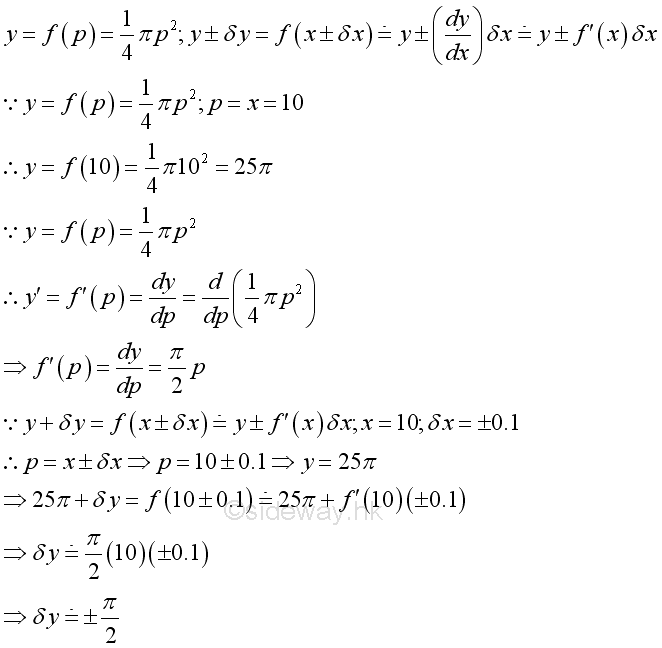### Change Determination Problem

In others word, the approximation determined by derivative can be the final change or the change. Therefore the derivative can also be used to estimate the initial value by determinating  the change.

For example, square of 24.9. Let function y be square function of a variable p. A known parameter of function is 25 and the knwon solution is 625. Therefore the solution of square of 24.9 is 620.01 and the approximation is: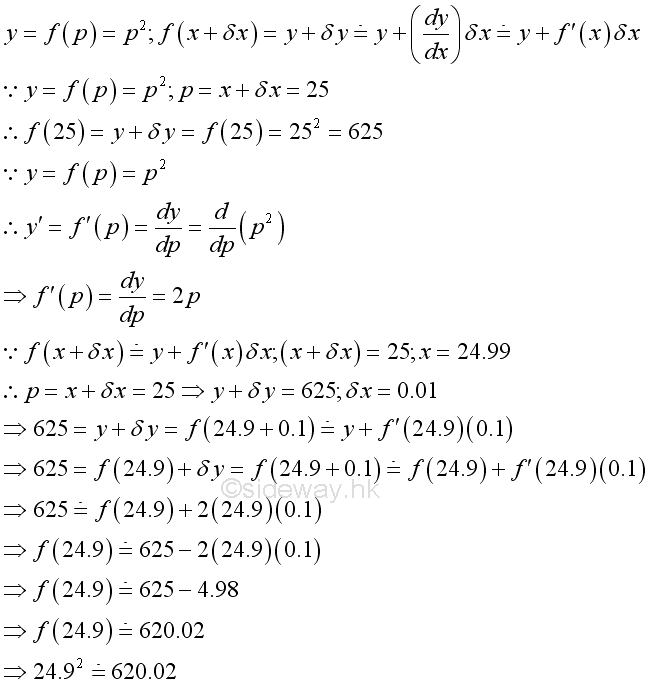ID: 111000013 Last Updated: 16/10/2011 Revision: 0 Ref:References

1. S. James, 1999
2. B. Joseph, 1978Home 5

Management

HBR 3

Information

Recreation

Culture

Chinese 1097

English 337

Computer

Hardware 149

Software

Application 187

Numeric 19

Programming

Web 757

CSS 1

HTML

Knowledge Base

OS 389

MS Windows

Knowledge

Mathematics

Algebra 20

Geometry 18

Calculus 67

Engineering

Mechanical

Rigid Bodies

Statics 92

Dynamics 37

Control

Physics

Electric 10